# Harmonic Mean Calculator

Use this harmonic mean calculator to easily calculate the Harmonic mean of a set of numbers.

Share calculator:

Embed this tool:
get code

## What is a harmonic mean?

The harmonic mean (archaic: subcontrary mean) is a specialized average of a set of numbers. It is one of the three Pythagorean means that provides the most accurate average. The harmonic mean is more complex to solve than the arithmetic, although they might seem similar at first. The difference between the two is that the harmonic mean calculates the reciprocal of the arithmetic mean of reciprocals.

The harmonic mean is largely used in situations dealing with quantitative data, such as finding the average of rates or ratios, due to the fact that it is not seriously affected by fluctuations.

## Harmonic mean formula

The formula for calculating the harmonic mean of a set of non-zero positive numbers is: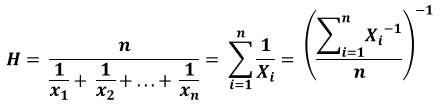where n is number of items and X1…X2 are the numbers from 1 to n.

To put it simply, all you need to do is divide the number of items in the set by the sum of their reciprocals. The above formula is what we use in this harmonic mean calculator.

## Calculating harmonic mean

If you're given the set of numbers: 3, 12, 20, 24, and have to find their harmonic mean, the first thing you should do is find a common denominator. It'll come in handy when you add up the reciprocals. In this case, all of the numbers are divisible by 120. Next up, find the sum of the reciprocals:

1/3 + 1/12 + 1/20 + 1/24 = 40/120 + 10/120 + 6/120 + 5/120 = 61/120.

As there are 4 items in the set, your final calculation should be:

4/ (61/120) = 4 x 120/61 = 7.87

An important note to be made is that you can't find the harmonic mean in a set of numbers, if one of them is 0.

Our harmonic mean calculator does the math for you! You just need to write down the number dataset, separating the items with a comma and a blank space.

## Weighted harmonic mean formula

The weighted harmonic mean is the reciprocal of the weighted average of the reciprocals of a weights dataset corresponding to a set of numbers. It is calculated with the formula: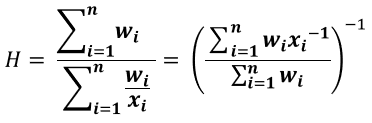## How to calculate the harmonic mean of two numbers

In specific cases where the set consists of two numbers only, you can calculate the harmonic mean using this formula: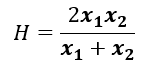. Even in this simple case it might be useful to turn to a calculator if the numbers are big or fractions.

The harmonic mean is in relation to the arithmetic mean (A = (X1 + X2)/2) and the geometric mean (G = √X1 x X2) in the following manner:

H = G2/A

Since in a set of real, non-negative numbers the arithmetic is always greater than the geometric mean, we can conclude that when n = 2, the harmonic mean will always be lesser in value or equal to the geometric mean (H ≤ G).

Another property of the Pythagorean means is that the geometric mean of two numbers is equal to the geometric mean on their arithmetic and harmonic averages (G = √A x H).

## Harmonic mean of three numbers

Three real, non-negative numbers A, G and H are respectively the average, geometric and harmonic means of three real, non-negative numbers if and only if this inequality is true: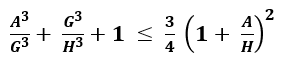## Example Usage in Finance

When averaging multiples where price is in the numerator, it's advisable to turn to the weighted harmonic mean. For example, if you want to calculate the price-earnings ratio with a weighted arithmetic average, the results would be biased upwards since it gives greater weight to higher data points. However, the weighted harmonic mean assigns equal weight to all data points , therefore providing the truest average.

Let's say you need to calculate the P/E stock index of 3 companies. The first one has market capitalization of \$10 billion and earnings of \$1 billion; the second has market capitalization of \$27 billion and earnings of \$3 billion; finally, the third company has market capitalization of \$100 million and earnings of \$2 million. You calculate the P/E ratio of the first, second, and third company to be respectively 10, 9, and 50. The index you have to calculate is made up of the three stocks: 60% invested in the first company, 25% in the second, and 15% invested in the third. Its P/E ratio, found using the weighted harmonic mean, is:

P/E = (0.6 + 0.25 + 0.15)/ 0.6/10 + 0.25/9 + 0.15/50 = 1/ 0.09 = 11.1

Now let's calculate it using the weighted arithmetic mean:

P/E = 0.6 x 10 + 0.25 x 9 + 0.15 x 50 = 15.75

Evidently, the weighted arithmetic average overestimates the index, thus giving you incorrect information.

## Example Usage in Physics

If you want to measure the average speed of a vehicle travelling a round trip, using the harmonic average you'll get the truest number. Let's say the distance from A to B is 120 km. It is known that the vehicle has travelled from point A to point B at a speed of 80 km/h, whereas the trip back from B to A was at a speed of 60 km/h. The equation for finding the average speed is:

Average speed = total distance/ total time

Average speed = 2 x 120/(120/80 + 120/60) = 240/3.5 = 68.6 km/h

You can verify the above result in the harmonic mean solver.

## Applications in Geometry

There are a few theorems regarding the usage of the harmonic mean in geometry:

• In each and any triangle, the harmonic mean of the three altitudes is equal to 1/3 of the radius (r) of the incircle;
• In a right-angled triangle with altitude (h) to the right angle from the hypotenuse, and legs a and b, the harmonic mean of a2 and b2 is twice as big as h2  ;
• If m and n are the sides of two inscribed squares in a right-angled triangle, then the harmonic mean of the squared hypotenuse c2 and m2 is twice as big as n2;
• If a point D lies on the minor arc BC of the circumcircle of an equilateral triangle ABC, it is at a distance m from B, and at a distance n from C.  The intersection of AD and BC is at a distance o from D. Therefore, the harmonic mean of m and n is twice as big as o ;
• The semi-latus rectum in an ellipse K is equal to the harmonic average of the minimum and maximum distances of K from a focus;
• In a trapezoid ABCD where AB and CD are parallel sides, the diagonals AC and BD intersect at point E. If point F lies on AD, and point G lies on BC, so that FEG is parallel to AB and CD, then FG is equal to the harmonic mean of AB and CD.

You can use this calculator to solve any of the above problems.

In the popular crossed ladders problem seen below, where two ladders are parallel to each other, one rises at a height A, the other at a height B. The two ladders cross at a height H from the ground. In this case, the harmonic mean of A and B is twice as big as H.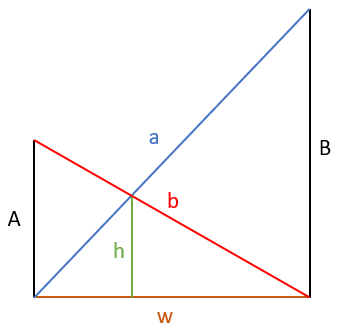## Application of the harmonic mean calculator in other sciences

Given the fact that the harmonic average is the most precise one, it is no surprise it has found its way into many sciences. For example, in computer sciences the harmonic mean is used to calculate the aggregate score for the evaluation of performance of machines, algorithms, and systems.

This average is useful in finding the exact densities and mass of particles in chemical analyses and nuclear physics tests.

In biology, more precisely in population genetics, the harmonic average is a means of calculating the fluctuation effects on generation size.

Students and researchers in these scientific disciplines often refer to a harmonic mean calculator when solving problems in their field.